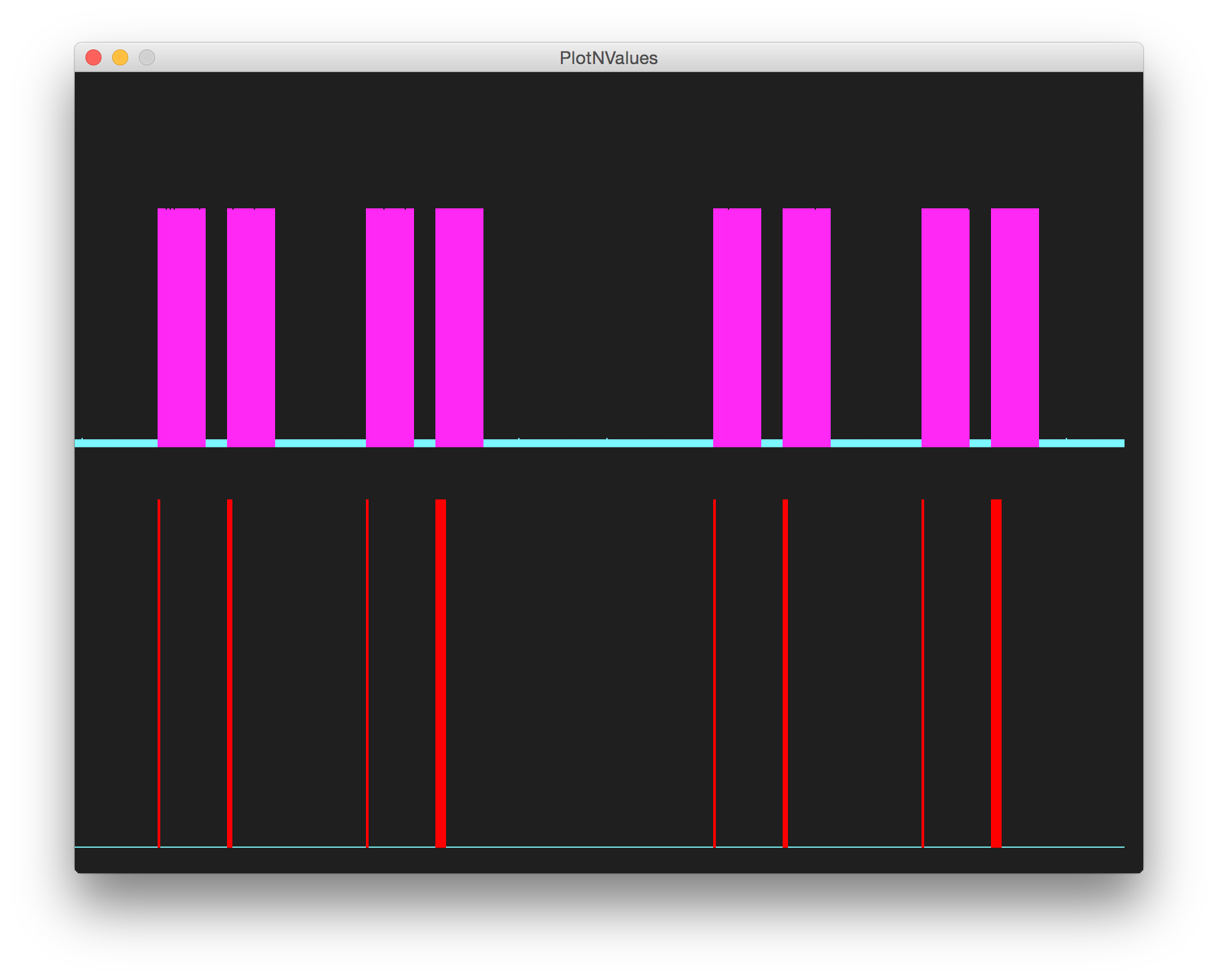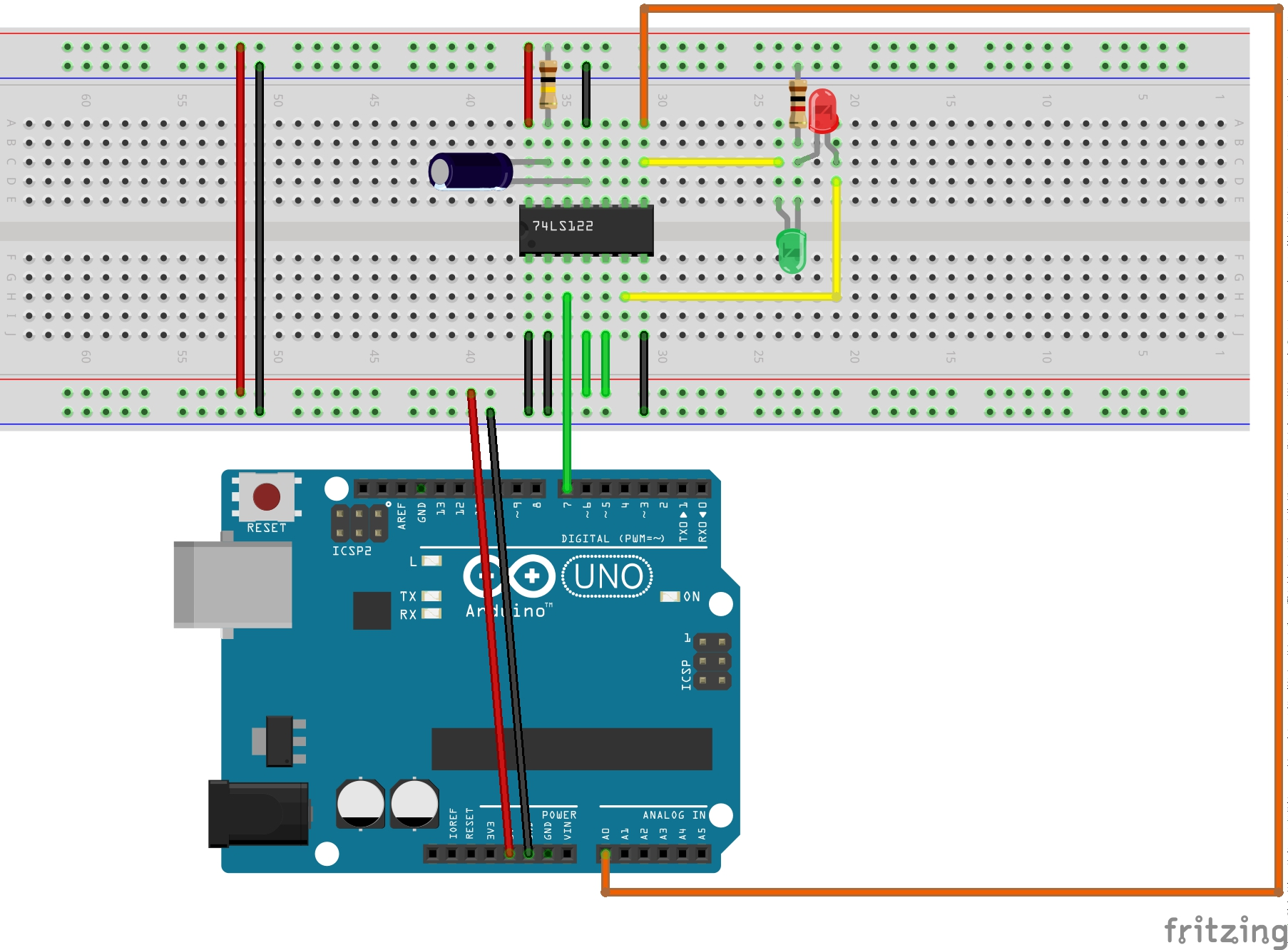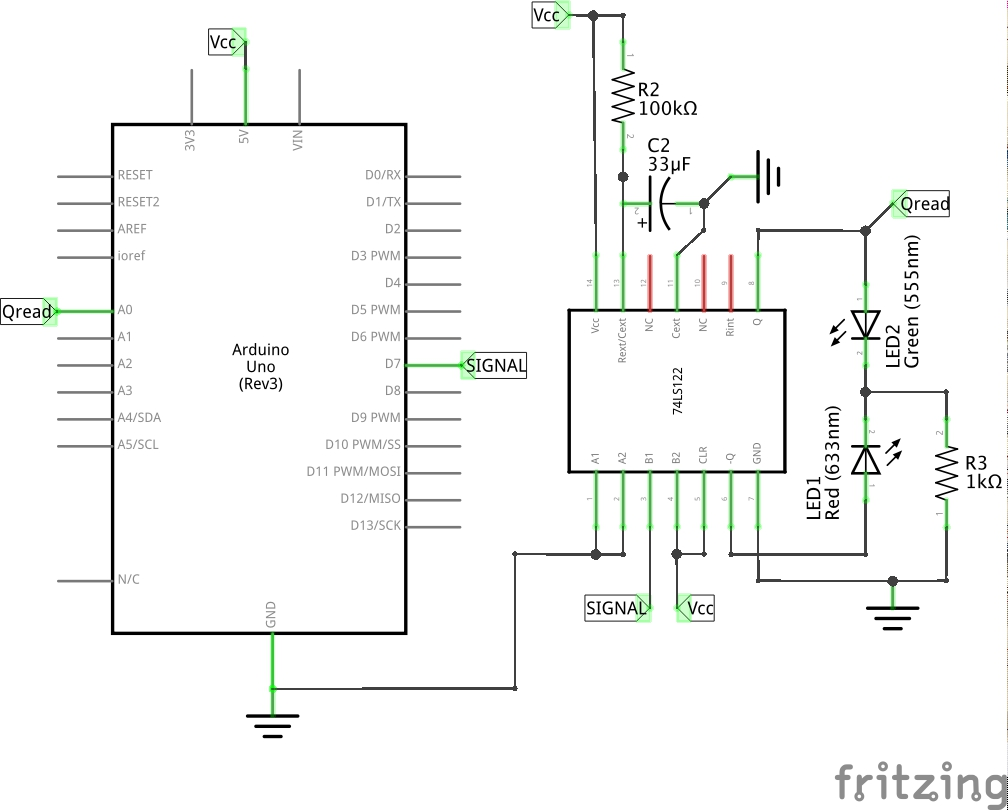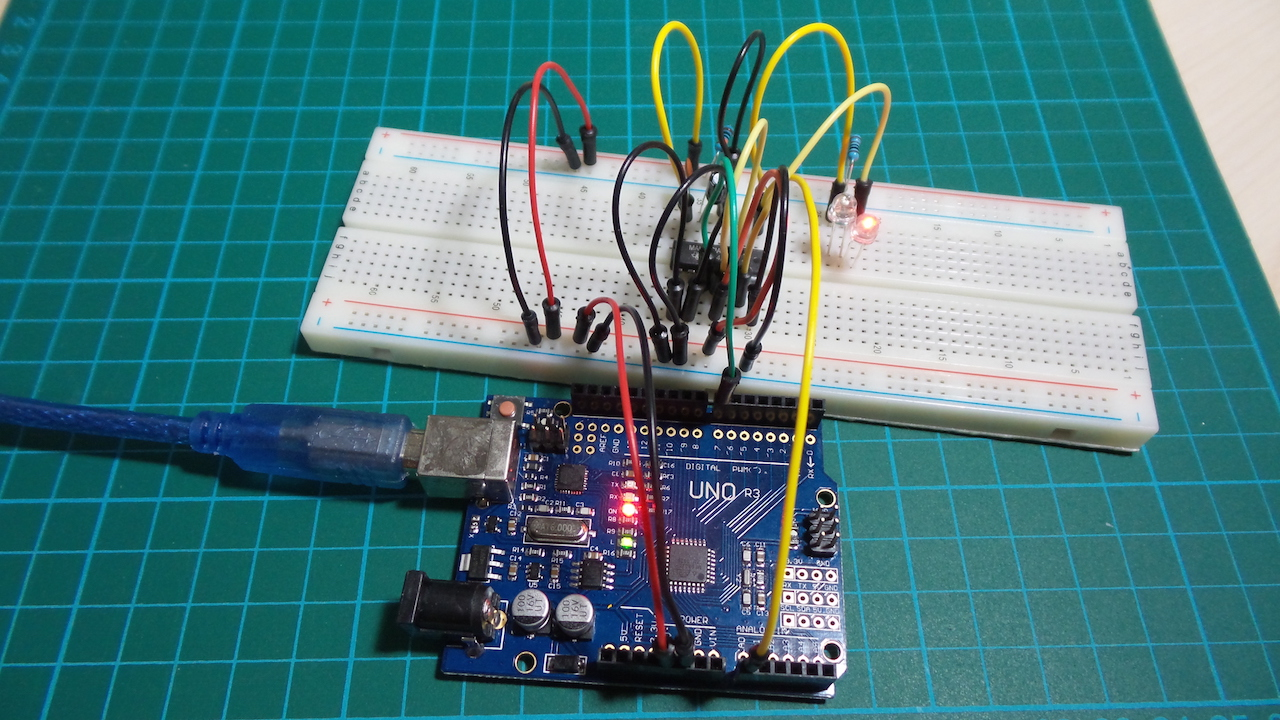# #076 MonostablePulseExtender

Use an Arduino to test the behaviour of a 74LS122 monostable with external timing configuration and plot the results.

The 74LS122 monostable multivibrator in this circuit has pulse width programmed by selection of external resistance and capacitance values R2 and C2. Pulse width is given as:

``````tW = K * R2 * C2
=> where K is nominally 0.45. If C2 is in μF and R2 is in kΩ then tW is in seconds
``````

For 33μF and 100kΩ then pulse width is 1.485s. This is intentionally large so that a visible pulse produced.

Once triggered, the basic pulse width may be extended by retriggering the gated low-level-active (A) or high-level-active (B) inputs, or be reduced by use of the overriding clear.

In this circuit an Arduino sends high-level-active (B) input pulses to trigger the monostable. LEDs are separately connected to output Q and Q complement, so they will toggle as the pulse goes high and low respectively.

The Arduino also reads output Q with an analog input pin and sends the data to PlotNValues (a simple Processing sketch). Here’s a trace of the behaviour. The lower trace is the trigger state, and the upper trace is the gate output.### Construction